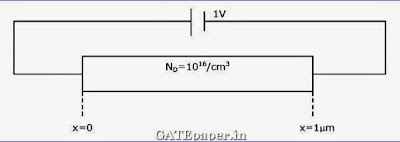### GATE 2010 ECE Video Solutions on EDC (Electronic Devices and Circuits)

1. At room temperature, a possible value for the mobility of electrons in the inversion layer of a silicon N channel MOSFET is (in cm2/volt-sec)
a. 450
b. 1350
c. 1800
d. 3600

2. The gate oxide in a CMOS process is preferably grown using
a. Wet oxidation
b. Dry oxidation
c. Epitaxial deposition
d. Ion implantation

3. In a uniformly doped BJT, assume that NE, NB and NC are the emitter, base and collector doping in atoms/cm3 respectively. If the emitter injection efficiency of the BJT is close to unity, which of the following conditions is TRUE?
a. NE = NB = NC
b. NE >> NB and NB > NC
c. NE = NB and NB < NC
d. NE < NB < NC

4. Compared to a PN junction with ND=NA=1014 per cm3, which one of the following statements is TRUE for a PN junction with NA=ND=1020 per cm3?
a. Reverse breakdown voltage is lower and depletion capacitance is lower
b. Reverse breakdown voltage is higher and depletion capacitance is lower
c. Reverse breakdown voltage is lower and depletion capacitance is higher
d. Reverse breakdown voltage is higher and depletion capacitance is higher

The silicon sample with unit cross sectional area shown below is in thermal equilibrium. The following information is given:
T = 300oK
Electron charge = 1.6x10-19 C
Thermal voltage = 26 mV
Electron mobility = 1350 cm2/volt-seci. The magnitude of the electric field at x = 0.5 µm is
a. 1 KV/cm
b. 5 KV/cm
c. 10 KV/cm
d. 26 KV/cm

ii. The magnitude of the electron drift current density at x = 0.5 µm is
a. 2.16x104 A/cm2
b. 1.08x104 A/cm2
c. 4.32x103 A/cm2
d. 6.48x102 A/cm2